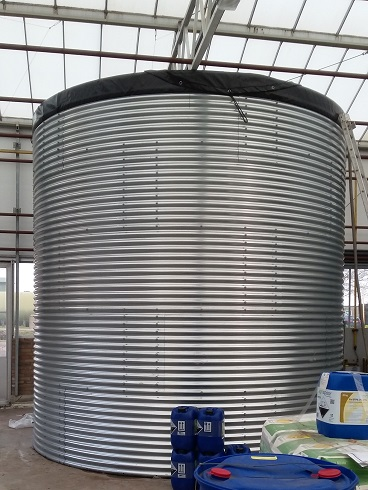Dijkgraaf 17
6921 RL Duiven
The Netherlands

# Water silo volume

## How to calculate the volume of a water silo?The volume of a water silo is calculated in the same way as that of a cylinder.

#### The formula is radius (diameter / 2) square x pi * = surface. Surface x length = volume

* Pi and mathematical constant with a fixed value of: 3.141592653 …

#### An example:

A water silo has a diameter of 5 meters and a height of 6 meters. The content is: 2,5² x 3.14 x 6m = 117.75 m³ The Silo has a volume of 117.75 cubic meters which equals 117750 liters of water. (1 cubic meter = 1000 liters)